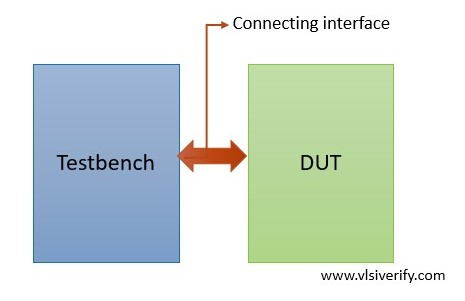Tutorials

Unlike Verilog that has module ports for communication, System Verilog provides an interface construct that simply contains a bundle of sets of signals. This encapsulates signals and communicates with design, testbench components.

1. In Verilog for the addition of new signals, it has to be manually changed everywhere that module has been instantiated. System Verilog made it easier to add new signals in the interface block for existing connections.
2. It has increased re-usability across the projects.
3. A set of signals can be easily shared across the components bypassing its handle.
4. It provides directional information (modports) and timing information (clocking blocks).
5. Interfaces can contain parameters, variables, functional coverage, assertions, tasks and functions.
6. Interfaces can contain procedural initial and always blocks and continuous assign statements.

Syntax:

``````interface <interface_name>;
...
endinterface``````## Writing an Interface

### A basic interface

``````interface bus (input clk);
logic [31:0] data;
logic en;
endinterface``````

### A parameterized interface

``````interface bus #(parameter WIDTH = 32)(input clk);
logic [WIDTH-1:0] data;
logic en;
endinterface``````

To understand how interfaces can be used in the environment, a full adder is constructed using two half adders. In case any new signal has to be added (like enable), it can be easily added to the interface without touching the port list in the module.

#### Full adder example without using an interface

Design Code:

``````module half_addr(input a, b, output so, co);
assign so = a^b;
assign co = a & b;
endmodule

module full_adder(input a, b, c, output s_out, c_out);
wire s0, c0, c1;
half_addr HA1 (a, b, s0, c0);
half_addr HA2 (s0, c, s_out, c1);

assign c_out = c0 | c1;
endmodule``````

TB Code:

``````module tb_top;
reg a, b, c;
wire s, c_out;

full_adder fa(a, b, c, s, c_out);

initial begin
\$monitor("a=%b b=%b c=%b, sum=%b, carry=%b",a,b,c,s,c_out);
a = 1; b = 0; c = 0;
#1;
a = 1; b = 0; c = 1;
#1;
a = 0; b = 1; c = 1;
end
endmodule``````

Output:

``````a=1 b=0 c=0, sum=1, carry=0
a=1 b=0 c=1, sum=0, carry=1
a=0 b=1 c=1, sum=0, carry=1``````

#### Full adder example using an interface

Design Code:

``````module half_addr(input a, b, output so, co);
assign so = a^b;
assign co = a & b;
endmodule

wire s0, c0, c1;
half_addr HA1 (inf.a, inf.b, s0, c0);
half_addr HA2 (s0, inf.c, inf.s_out, c1);

endmodule``````

TB Code:

``````interface fa_if;
logic a, b, c;
logic s_out, c_out;
endinterface

module tb_top;
fa_if inf();

initial begin
\$monitor("a=%b b=%b c=%b, sum=%b, carry=%b",inf.a,inf.b,inf.c,inf.s_out,inf.c_out);
inf.a = 1; inf.b = 0; inf.c = 0;
#1;
inf.a = 1; inf.b = 0; inf.c = 1;
#1;
inf.a = 0; inf.b = 1; inf.c = 1;
end
endmodule``````

Output:

``````a=1 b=0 c=0, sum=1, carry=0
a=1 b=0 c=1, sum=0, carry=1
a=0 b=1 c=1, sum=0, carry=1``````

### Interface example using clk

Design Code:

``````module multiplier(mult_if inf);

always@(posedge inf.clk or posedge inf.reset) begin
if(inf.reset) begin
inf.out <= 0;
inf.ack <= 0;
end
else if(inf.en) begin
inf.out <= inf.a * inf.b;
inf.ack <= 1;
end
else inf.ack <= 0;
end
endmodule``````

TB Code:

``````interface mult_if (input logic clk, reset);
logic [7:0] a, b;
logic [15:0] out;
logic en;
logic ack;
endinterface

module tb_top;
bit clk;
bit reset;

always #2 clk = ~clk;

initial begin
clk = 0;
reset = 1;
#2;
reset = 0;
end

mult_if inf(clk, reset);
multiplier DUT(inf);

initial begin
#5;
inf.a = 'd5; inf.b = 'd6;
inf.en = 1;
#10 inf.en = 0;
wait(inf.ack);
\$display("%0t: a=%d b=%d, out=%d", \$time, inf.a,inf.b,inf.out);

#25;
inf.a = 'd20; inf.b = 'd7;
#5ns inf.en = 1;
#6 inf.en = 0;
wait(inf.ack);
\$display("%0t: a=%d b=%d, out=%d", \$time, inf.a,inf.b,inf.out);

#25;
inf.a = 'd10; inf.b = 'd4;
#6ns inf.en = 1;
#5 inf.en = 0;
wait(inf.ack);
\$display("%0t: a=%d b=%d, out=%d", \$time, inf.a,inf.b,inf.out);
#10;
\$finish;
end

initial begin
\$dumpfile("dump.vcd"); \$dumpvars;
end
endmodule``````

Output:

``````15: a=  5 b=  6, out=   30
51: a= 20 b=  7, out=  140
87: a= 10 b=  4, out=   40``````

### Why is the logic data type used for the signal declaration or why bit data type is not used?

A bit data type is a 2-state data type that can have either 0 or 1 value. For incorrect RTL behavior like X or Z testbench would have sampled as 0. So, it needs a 4-state data type that can capture all 4 states 0, 1, X, or Z.

System Verilog Tutorials